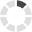### Site InformationLoading... Please wait...

# Battery Basics 101

### Amps and Volts: Battery Basics?

This is a back to basic article on understanding a batteries electrical ratings. Let's take a real question from a real person:

"I have a sony clie PEG-NR70V/U PDA. The battery that you show that would fit my unit is 1200 mah and 3.7 volts. On the back of my PDA it says 800 mAh and the volts is 5.2. Is the battery that you show for my unit the same?"

This is a great question and is actually quite common and so it is wise that we gain a better understanding of what the ratings mean.

All PDA batteries have an electrical specifications that include its volt and milliAmp hour rating. These terms are abbreviated as we see in the following example: 3.7 V, 1600 mAh.

Volts - or V - are an electrical measure of energy potential. You can think of it as the pressure being exerted by all the electrons of a PDA Batteries negative terminal as they try to move to the positive terminal.

Amps - or A - is an abbreviation of Ampere, a 19th century French scientist who was a pioneer in electricity research. Amps measure the volume of electrons passing through a wire in a one second. One Amp equals 6.25 x 1018 electrons per second.

Amp hours - or Ah - measures capacity. That is what is ultimately important to consumers as it is the capacity or amp hours that tells us how long we can expect a battery to deliver a charge before it runs out. As with all metric measurements, Amps can be divided into smaller (or larger) units by adding a prefix, in this case by adding an "m" to the amp hour we are renaming the amp hour to milli amp hour: mAh.

In the case of PDA Batteries, a milliAmp hour (mAh) is most commonly used notation system. Note that 1000 mAh is the same a 1 Ah. (Just as 1000mm equals 1 meter.) Note that Amp hours do not dictate the flow of electrons at any given moment, that is the role of volts. PDA batteries with a 1 Amp hour rating could deliver ½ Amp of current for 2 hours, or they could provide 2 Amps of current for ½ hour.

Typically, PDA Batteries will use 1 to 3 Amps per hour, depending on the model's processor speed, screen size, screen brightness adjustment, usage, and other factors.

Some of our batteries will have higher amp-hour ratings than the original battery found in your device and will not cause any incompatibilities. It is actually good because you are getting greater capacity.

Volts on the other hand have to be within a nominal range (reasonable range) of each other. Manufacturers rate a voltage cell, which then becomes the nominal voltage, historically with a 3.6V while others picked 3.7V to name the cell. The functionality and performance of either cell is identical and cannot be differentiated by the device. The explanation above applies to a single Li-Ion cell in series. When a battery has two or more Li-Ion cells in series, the voltage is multiplied by the number of cells in series.

Now back to the question we began with.....

"I have a sony clie PEG-NR70V/U PDA. The battery that you show that would fit my unit is 1200 mah and 3.7 volts. On the back of my PDA it says 800 mAh and the volts is 5.2. Is the battery that you show for my unit the same?"

To understand the answer to the question let's look at a math formula for the complete formula of Watts....

Watts = Volts x Amps x k(one unit length of wire)

Since the length of your PDA's wire inside the battery casing is difficult to know (unless you open the casing) let's just use a more common formula for Watts: (NOTE: DO NOT OPEN YOUR BATTERY'S CASING, EXTREME HARM WILL COME TO YOU)

Watts = Volts x Amps

Now the orignial Clie battery came with 800 mAh and the volts is 5.2. So the Watts would be:

41.6= 5.2 x 8

The Clie battery on our page is 3.7 V and 1200 mAh. So the Watts would be:

44.4= 3.7 x 12

Remember that I'm 1200 mAh is the same as 12 Ah and 800 mAh is the same as 8 Ah

So as we discussed early a nominal range is inherent in voltage ratings and slight variations in voltage generally do not impact the performance of your PDA. We see this all the time with universal and external batteries. The original battery might be specified at 10.8 Volts, but customers using a universal part can operate their laptop safely at either the 10 or 11 Volt setting.

There are no products in this category.